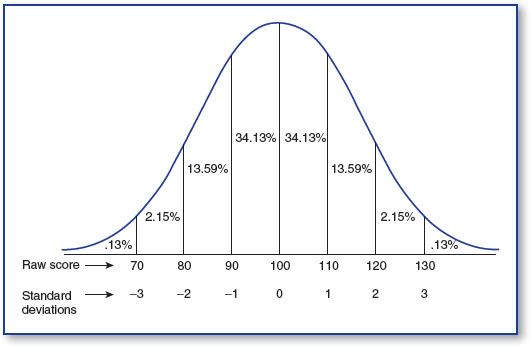In an increasingly data-driven world, it is more important than ever for students as well as professionals to better understand basic statistical concepts. 100 Questions (and Answers) About Statistics addresses the essential questions that students ask about statistics in a concise and accessible way. It is perfect for instructors, students, and practitioners as a supplement to more comprehensive materials, or as a desk reference with quick answers to the most frequently asked questions.

# What Is the Relationship Between z Scores and the Normal Curve?

### What Is the Relationship Between z Scores and the Normal Curve?

The normal curve has the three characteristics that we already mentioned: the mean, median, and the mode being equal to one another; both sides being symmetrical; and the tails never touching the x-axis.

But it has another set of characteristics that are very important to our discussion of z scores and the idea of inference and the use of inferential statistics.

The normal curve can be divided into segments, as you see in Figure 72.1, and you can see that a specific percentage of outcomes falls within each of these areas.

### Figure 72.1 The normal curve and associated percentagesFor example, between the mean and 1 z score (or standard deviations), you will find 34.14% of ...

•••